## Introduction

In this vignette, we will demonstrate how to calculate and plot a range of performance metrics for a clinical risk score across demographic groups. We will use both simulated and real data. Please see our manuscript for further details.

Our main risk score of interest is the SPARRA score [@sparrav3], which predicts emergency hospital admission in the coming year given data from electronic health records for the majority of the population of Scotland. We analyse the currently-used SPARRA version 3 score but this package and vignette also allows for analysis of the in-development SPARRA version 4 score [@sparrav4].

## Simulation of data

Raw data for the SPARRA version 3 score comprises individual-level medical records and is private. In order to demonstrate computation of performance metrics, we will begin by simulating semi-realistic data for 10,000 individuals.


library(SPARRAfairness)
library(ranger)

# Get data
data(all_data)
data(decomposition_matrix)

# Set random seed
seed=463825
set.seed(seed)

# Simulate data
pop_data=sim_pop_data(10000)

# First few rows
#>   age  sexM raceNW prevAdm SIMD urban_rural mainland_island target id
#> 1  39  TRUE  FALSE       1    8       FALSE           FALSE      1  1
#> 2  46 FALSE  FALSE       1   10       FALSE           FALSE      1  2
#> 3  22  TRUE  FALSE       0    9       FALSE           FALSE      0  3
#> 4  77 FALSE  FALSE       0   10       FALSE           FALSE      1  4
#> 5  88  TRUE  FALSE       6   10        TRUE           FALSE      1  5
#> 6  74 FALSE  FALSE       9    5       FALSE           FALSE      1  6
#>                  reason
#> 1                 Blood
#> 2                   Ear
#> 3                  <NA>
#> 4           Circulatory
#> 5 Died.of.Genitourinary
#> 6            Congenital


## Fit risk score

We will fit a risk score to this data using a random forest, using the ranger package with default settings (500 trees). We will use scores generated from a model fitted directly to our simulated data, which will be somewhat overfitted, but for our purposes will be adequate.


# Fit model
sim_model=ranger(
data=pop_data)

# Model predictions
score=predict(sim_model,pop_data)$predictions # Affix to pop_data pop_data$score=score


## Define groups

We will define groups based on urban/rural postcode: 1 will be urban, 2 rural

group1=which(pop_data$urban_rural==FALSE) group2=which(pop_data$urban_rural==TRUE)


## Generate metrics

We will look at threshold score values evenly spaced between 0 and 1.

score_cutoffs=seq(0,1,length=101)


### Score distributions

We begin with distribution of scores, or demographic parity [@calders09,@zliobaite15]:

dem_par=demographic_parity(pop_data$score,group1,group2,cutoffs=score_cutoffs)  ### Counterfactual score distributions We now consider the distribution of scores of a counterfactual set of individuals, distributed as though they were urban residents, but are rural. We take age and sex as downstream of urban/rural status. Essentially we isolate causes of differential score distributions to those attributable to age and sex. We do this by generating a 'counterfactual sample' from our data. Please see the examples for counterfactual_yhat for a more in-depth look at what we mean by counterfactuals and what this funtion does.  # Counterfactual sample (samples are rural, but 'resemble' urban samples) cf_rural_ids=counterfactual_yhat(dat=pop_data,X=c("age","sexM"), x=NULL,G="urban_rural", g=FALSE,gdash=TRUE, excl=c("id","score","target")) # Put together into data frame cf_pop_data=rbind(pop_data[group1,],pop_data[cf_rural_ids,]) cf_group1=which(cf_pop_data$urban_rural==FALSE);
cf_group2=which(cf_pop_data$urban_rural==TRUE) cf_dem_par=demographic_parity(cf_pop_data$score,cf_group1,
cf_group2,cutoffs=score_cutoffs)


### Score performance

We then look at performance in each group, first looking at ROC curves. In generating ROC and PRC curves, the functions getroc and getprc return 100 equally-spaced points along the curve, rather than the full curve which would have 10,000 points.

roc1=getroc(pop_data$target[group1],pop_data$score[group1])
#> Warning in regularize.values(x, y, ties, missing(ties), na.rm = na.rm):
#> collapsing to unique 'x' values
roc2=getroc(pop_data$target[group2],pop_data$score[group2])
#> Warning in regularize.values(x, y, ties, missing(ties), na.rm = na.rm):
#> collapsing to unique 'x' values


then PR curves

prc1=getprc(pop_data$target[group1],pop_data$score[group1])
prc2=getprc(pop_data$target[group2],pop_data$score[group2])


then calibration curves [@brocker07]:

cal1=getcal(pop_data$target[group1],pop_data$score[group1])
cal2=getcal(pop_data$target[group2],pop_data$score[group2])


### False omission and false discovery rates

We assess false omission rate. Where $$Y$$ is target, $$\hat{Y}$$ is score, $$G$$ is group, this is $$P(Y=1|\hat{Y} \leq c,G=g)$$, considered across groups $$g$$ and cutoffs $$c$$. We compute this by defining a specifications vector (see documentation of function group_fairness):

spec_for=c(NA,1,NA,0,NA,1)
for_12=group_fairness(spec_for,pop_data$score,pop_data$target,
group1,group2,cutoffs = score_cutoffs)


We then assess false discovery rate: $$P(Y=0|\hat{Y} > c,G=g)$$, considered across groups $$g$$ and cutoffs $$c$$.

spec_fdr=c(NA,0,NA,1,NA,1)
fdr_12=group_fairness(spec_fdr,pop_data$score,pop_data$target,
group1,group2,cutoffs = score_cutoffs)


Since false discovery and false positive rates might be influenced by different distributions of age and sex between groups, we adjust for these. We define a categorisation by age and sex

cat_12=paste0(pop_data$age,"_",pop_data$sexM)
for_adjusted_12=adjusted_for(pop_data$score,pop_data$target,cat_12,
group1,group2,cutoffs=score_cutoffs,nboot=10)
fdr_adjusted_12=adjusted_fdr(pop_data$score,pop_data$target,cat_12,
group1,group2,cutoffs=score_cutoffs,nboot=10)


## Plotting data

We now plot the output of these computations. We also plot similar figures for real data.

We set some general settings

alpha=0.05 # We will plot 1-alpha confidence intervals
q=-qnorm(alpha/2) # Multiply standard errors by this to get half CI width
highlight_value=0.5 # Highlight what happens at a cutoff of 0.5/50%
colour_scheme <- phs_colours(c("phs-blue",
"phs-purple",
"phs-magenta")) # set colour scheme


### Score distributions

We plot demographic parity on a log scale. Note score is given as a percentage.

obj_list=list(
list(x=score_cutoffs,y=dem_par[1,],ci=q*dem_par[2,]),
list(x=score_cutoffs,y=dem_par[3,],ci=q*dem_par[4,])
)
groupmetric_2panel(obj_list,
labels=c("Urban","Rural"),
col=phs_colours(c("phs-blue","phs-magenta")),
ci_col=phs_colours(c("phs-blue","phs-magenta")),
yrange=c(0,1),
lpos="topleft",
yrange_lower=c(-0.2,0.2),
highlight=highlight_value,
logscale=TRUE)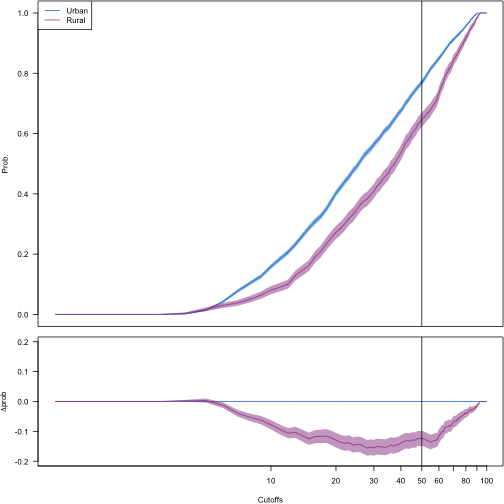An equivalent plot for real data:

obj=all_data$dp_v3_Urban_rural_all obj_list=list( list(x=exp(obj$xx),y=obj$yA,ci=obj$cA),
list(x=exp(obj$xx),y=obj$yB,ci=obj$cB) ) groupmetric_2panel(obj_list, labels=c("Urban","Rural"), col=phs_colours(c("phs-blue","phs-magenta")), ci_col=phs_colours(c("phs-blue","phs-magenta")), yrange=c(0,1), lpos="topleft", yrange_lower=c(-0.2,0.2), highlight=highlight_value, logscale=TRUE)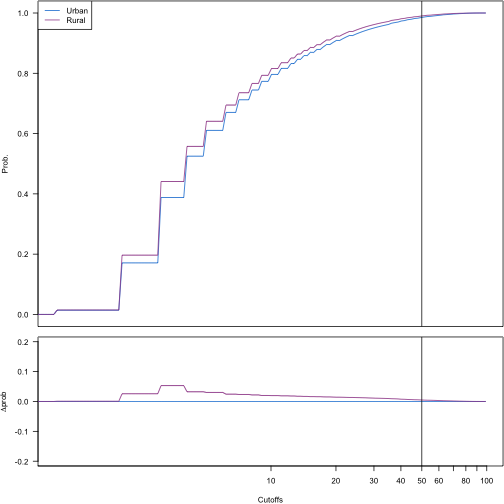### Counterfactual score distributions We plot counterfactual score distributions in a similar way obj_list=list( list(x=score_cutoffs,y=cf_dem_par[1,],ci=q*cf_dem_par[2,]), list(x=score_cutoffs,y=cf_dem_par[3,],ci=q*cf_dem_par[4,]) ) groupmetric_2panel(obj_list, labels=c("Urban","Rural"), col=phs_colours(c("phs-blue","phs-magenta")), ci_col=phs_colours(c("phs-blue","phs-magenta")), yrange=c(0,1), lpos="topleft", yrange_lower=c(-0.2,0.2), highlight=highlight_value, logscale=TRUE)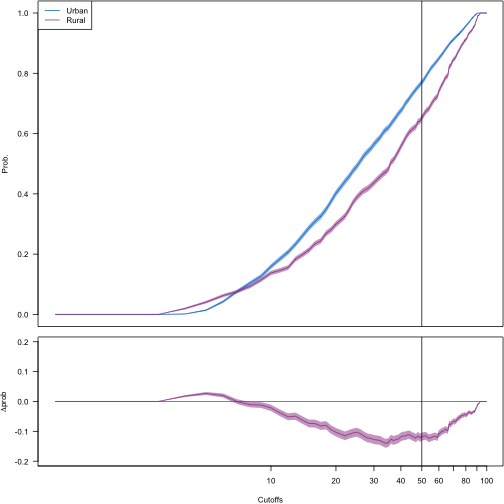An equivalent plot for real data: obj=all_data$counterfactual_dp_v3_Urban_rural

obj_list=list(
list(x=exp(obj$xx),y=obj$yA,ci=obj$cA), list(x=exp(obj$xx),y=obj$yB,ci=obj$cB)
)
groupmetric_2panel(obj_list,
labels=c("Urban","Rural"),
col=phs_colours(c("phs-blue","phs-magenta")),
ci_col=phs_colours(c("phs-blue","phs-magenta")),
yrange=c(0,1),
lpos="topleft",
yrange_lower=c(-0.2,0.2),
highlight=highlight_value,
logscale=TRUE)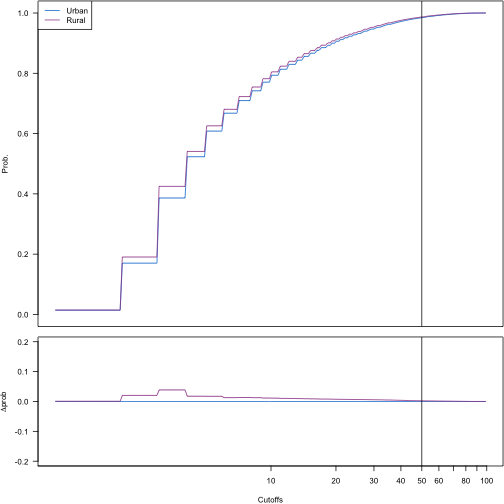### Score performance

We plot ROC curves, PR curves and calibration curves for both groups

roc_2panel(list(roc1,roc2),
labels = c("Urban","Rural"),
col=colour_scheme,
highlight=highlight_value,
yrange_lower=c(-0.2,0.2))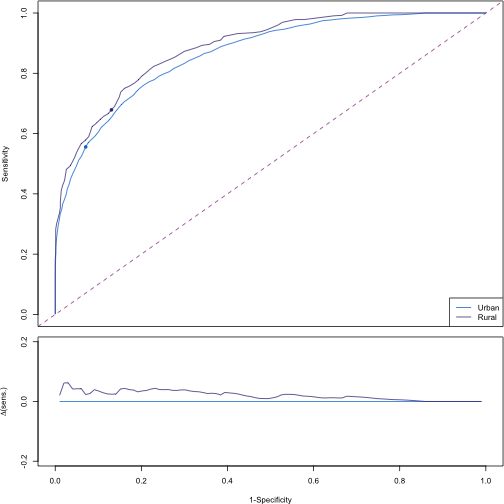For real data:

roc_2panel(all_data$roc_v3_Urban_rural[1:3], labels = c("Overall","Urban","Rural"), col=colour_scheme, highlight=highlight_value, yrange_lower=c(-0.2,0.2))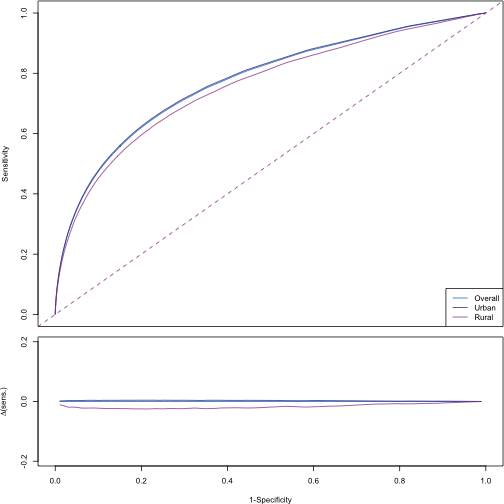We now plot PR curves: prc_2panel(list(prc1,prc2), labels = c("Urban","Rural"), col=colour_scheme, highlight=highlight_value, yrange_lower=c(-0.2,0.2))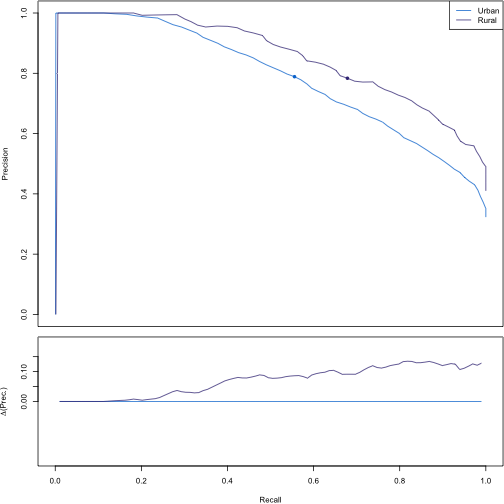For real data: prc_2panel(all_data$prc_v3_Urban_rural[1:3],
labels = c("Overall","Urban","Rural"),
col=colour_scheme,
highlight=highlight_value,
yrange_lower=c(-0.2,0.2))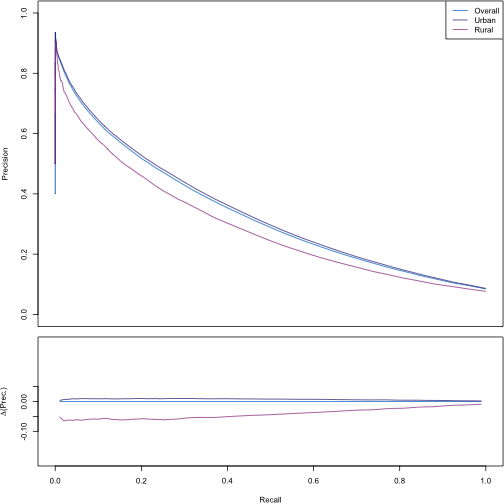Finally, we plot calibration curves (reliability diagrams):

cal_2panel(list(cal1,cal2),
labels = c("Urban","Rural"),
col=colour_scheme,
highlight=highlight_value,
yrange_lower=c(-0.2,0.2))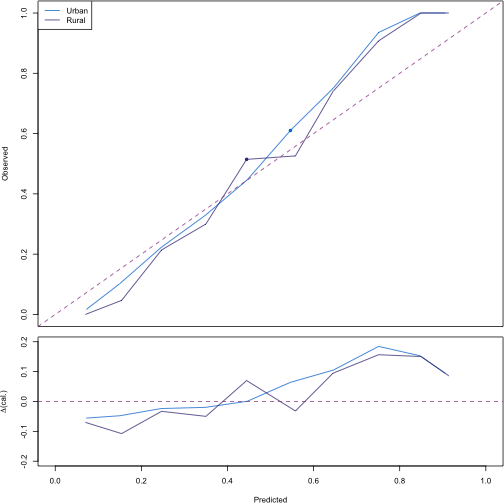For real data:

cal_2panel(all_data$cal_v3_Urban_rural[1:3], labels = c("Overall","Urban","Rural"), col=colour_scheme, highlight=highlight_value, yrange_lower=c(-0.2,0.2))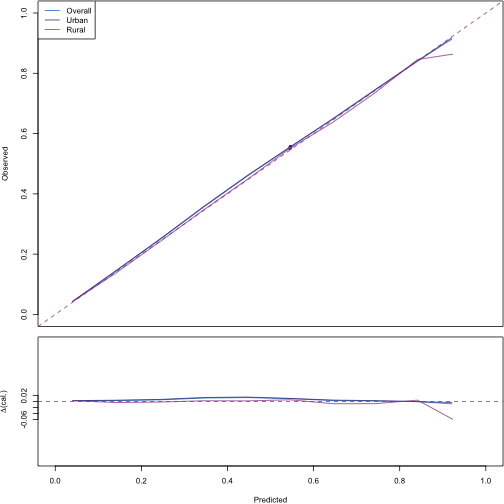### False omission and false discovery rates We plot raw false omission rates as follows: obj_list=list( list(x=score_cutoffs,y=for_12[1,],ci=for_12[2,]), list(x=score_cutoffs,y=for_12[3,],ci=for_12[4,]) ) groupmetric_2panel(obj_list, labels=c("Urban","Rural"), col=phs_colours(c("phs-blue","phs-magenta")), ci_col=phs_colours(c("phs-blue","phs-magenta")), yrange=c(0,0.5), lpos="topleft", yrange_lower=c(-0.1,0.1), highlight=highlight_value)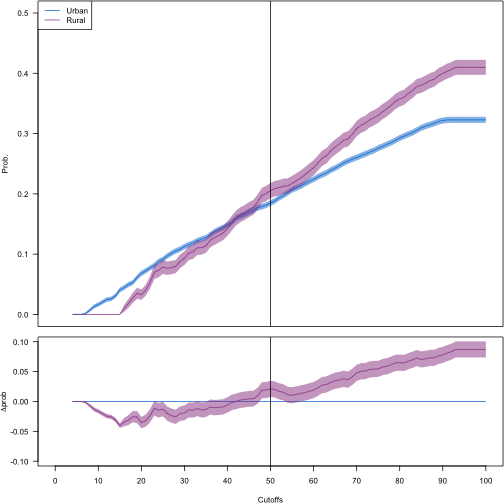and false discovery rates as follows: obj_list=list( list(x=score_cutoffs,y=fdr_12[1,],ci=fdr_12[2,]), list(x=score_cutoffs,y=fdr_12[3,],ci=fdr_12[4,]) ) groupmetric_2panel(obj_list, labels=c("Urban","Rural"), col=phs_colours(c("phs-blue","phs-magenta")), ci_col=phs_colours(c("phs-blue","phs-magenta")), yrange=c(0,1), lpos="topleft", yrange_lower=c(-0.1,0.1), highlight=highlight_value)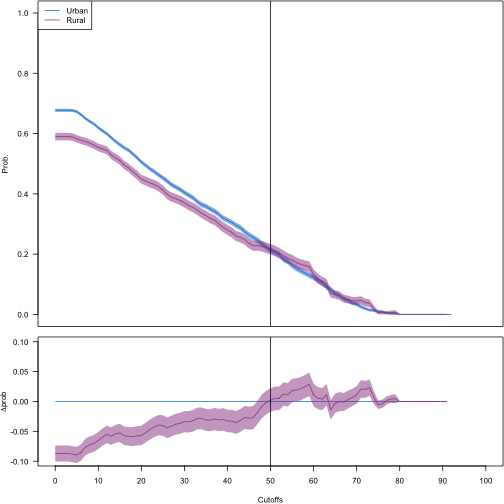For real data, we plot false omission rates as follows: obj=all_data$forp_v3_Urban_rural_all

obj_list=list(
list(x=obj$cutoffs,y=obj$p_AA,ci=obj$ci_AA), list(x=obj$cutoffs,y=obj$p_BB,ci=obj$ci_BB)
)
groupmetric_2panel(obj_list,
labels=c("Urban","Rural"),
col=phs_colours(c("phs-blue","phs-magenta")),
ci_col=phs_colours(c("phs-blue","phs-magenta")),
yrange=c(0,0.1),
lpos="topleft",
yrange_lower=c(-0.02,0.02),
highlight=highlight_value)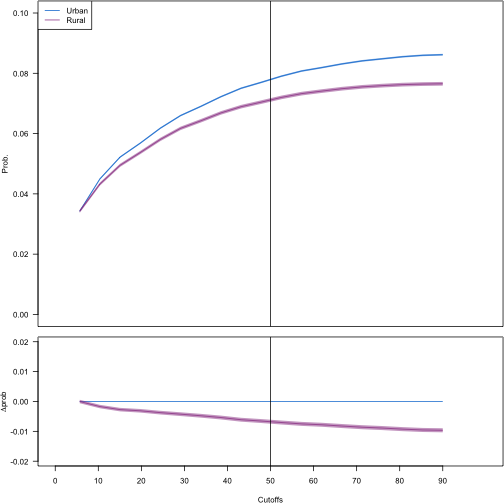and false discovery rates as follows:

obj=all_data$fdrp_v3_Urban_rural_all obj_list=list( list(x=obj$cutoffs,y=obj$p_AA,ci=obj$ci_AA),
list(x=obj$cutoffs,y=obj$p_BB,ci=obj$ci_BB) ) groupmetric_2panel(obj_list, labels=c("Urban","Rural"), col=phs_colours(c("phs-blue","phs-magenta")), ci_col=phs_colours(c("phs-blue","phs-magenta")), yrange=c(0,1), lpos="topright", yrange_lower=c(-0.1,0.1), highlight=highlight_value)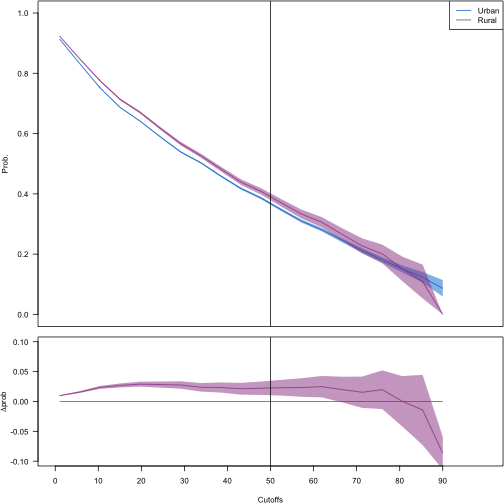We plot adjusted false omission rates as follows: obj_list=list( list(x=score_cutoffs,y=for_adjusted_12[1,],ci=for_adjusted_12[2,]), list(x=score_cutoffs,y=for_adjusted_12[3,],ci=for_adjusted_12[4,]) ) groupmetric_2panel(obj_list, labels=c("Urban","Rural"), col=phs_colours(c("phs-blue","phs-magenta")), ci_col=phs_colours(c("phs-blue","phs-magenta")), yrange=c(0,0.5), lpos="topleft", yrange_lower=c(-0.1,0.1), highlight=highlight_value)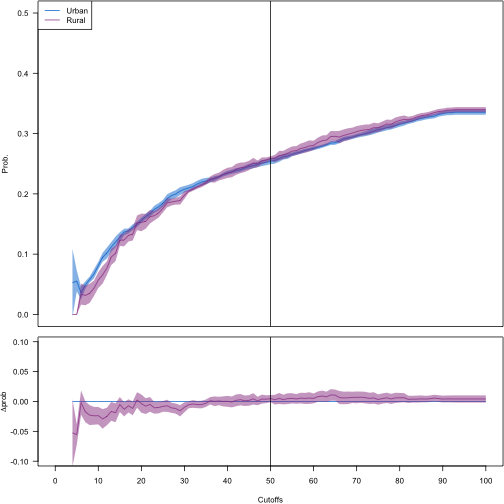and false discovery rates as follows: obj_list=list( list(x=score_cutoffs,y=fdr_adjusted_12[1,],ci=fdr_adjusted_12[2,]), list(x=score_cutoffs,y=fdr_adjusted_12[3,],ci=fdr_adjusted_12[4,]) ) groupmetric_2panel(obj_list, labels=c("Urban","Rural"), col=phs_colours(c("phs-blue","phs-magenta")), ci_col=phs_colours(c("phs-blue","phs-magenta")), yrange=c(0,1), lpos="topleft", yrange_lower=c(-0.1,0.1), highlight=highlight_value)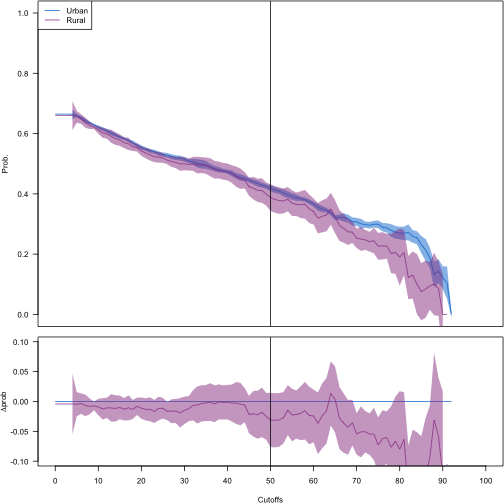For real data, we plot false omission rates as follows: obj=all_data$forp_adjusted_v3_Urban_rural

obj_list=list(
list(x=obj$cutoffs,y=obj$p_AA,ci=obj$ci_AA), list(x=obj$cutoffs,y=obj$p_BB,ci=obj$ci_BB)
)
groupmetric_2panel(obj_list,
labels=c("Urban","Rural"),
col=phs_colours(c("phs-blue","phs-magenta")),
ci_col=phs_colours(c("phs-blue","phs-magenta")),
yrange=c(0,0.1),
lpos="topleft",
yrange_lower=c(-0.02,0.02),
highlight=highlight_value)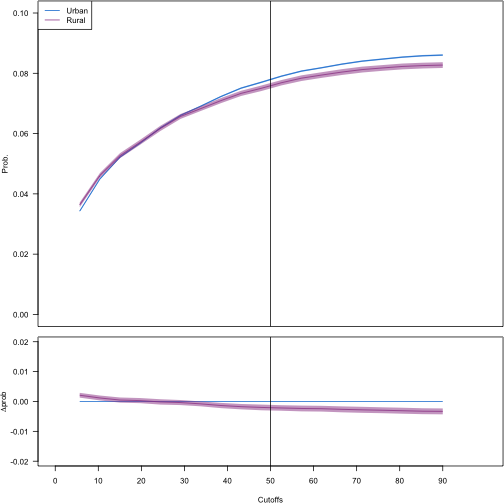and false discovery rates as follows:

obj=all_data$fdrp_adjusted_v3_Urban_rural obj_list=list( list(x=obj$cutoffs,y=obj$p_AA,ci=obj$ci_AA),
list(x=obj$cutoffs,y=obj$p_BB,ci=obj$ci_BB) ) groupmetric_2panel(obj_list, labels=c("Urban","Rural"), col=phs_colours(c("phs-blue","phs-magenta")), ci_col=phs_colours(c("phs-blue","phs-magenta")), yrange=c(0,1), lpos="topright", yrange_lower=c(-0.1,0.1), highlight=highlight_value)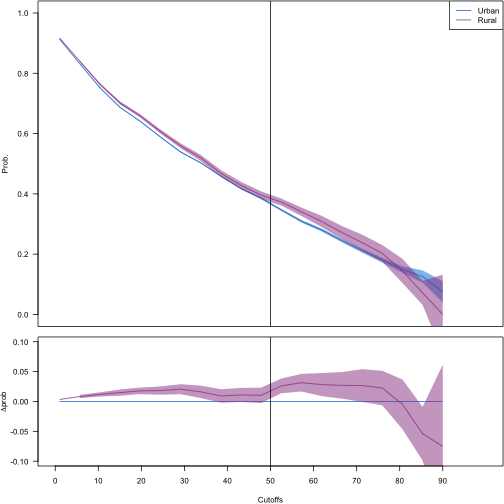## Decompose FOR by admission type Amongst individuals who have had a hospital admission, we may wish to probe the distribution of reasons for admission. This distribution of reasons may be expected to change across demographic groups. We will use a score threshold of 50% or 0.5. We begin by decomposing admissions by type and score quantile. See documentation for plot_decomp() for details of what we produce. cutoff=0.5 # 10% risk score threshold decomp_matrices=dat2mat(pop_data, score=pop_data$score,
group1=which(pop_data$urban_rural==0), group2=which(pop_data$urban_rural==1),
nquant=20)
plot_decomp(decomp_matrices$matrix1, decomp_matrices$matrix2,
threshold=cutoff,
labels=c("Urban","Rural"))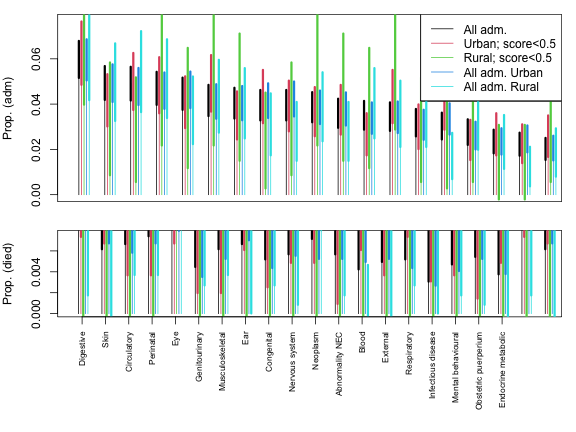We now plot the corresponding figure for real data:

cutoff=0.5 # 10% risk score threshold
names_group1=paste0("v3_Urban_q",1:20)
names_group2=paste0("v3_Rural_q",1:20)
decomp1=decomposition_matrix[names_group1,]
decomp2=decomposition_matrix[names_group2,]
plot_decomp(decomp1,
decomp2,
threshold=cutoff,
labels=c("Urban","Rural"))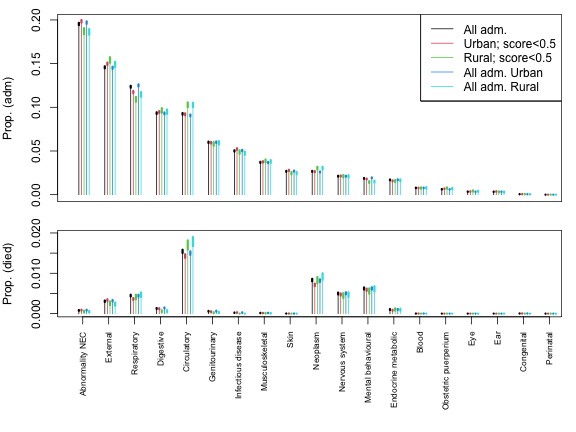## Plot a diagram

Finally, if we so wish, we may illustrate a proportion as a group of people of various colours. We may plot an individual human figure as follows:

plot(0,type="n",bty="n",ann=F,xlim=c(-1,1)/2,ylim=c(-1,1)/2)
drawperson(0,0,col="blue",border="gray",lwd=3)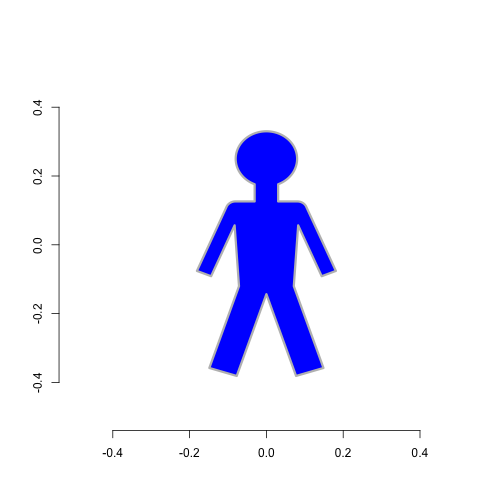and illustrate a proportion as follows. Human igures in the 'ci' region are coloured with a gradient between colours.

drawprop(0.3,ci=c(0.2,0.4),col1 = "blue",col2="gray")